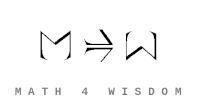文章 发现 ms@ms.lt +370 607 27 665 My work is in the Public Domain for all to share freely. 读物 书 影片 维基百科 Software Upload Investigation: Specify the category theory that grounds Grothendieck's six operations. Investigate How do the six operations match the six criteria? Analyze the six operations as functors, considering their domains and codomains. Use insights from the Hopf algebra (the duality of multiplication and comultiplication) to try to relate the six bases of symmetric functions to the six operations. How does tensor product join subsystems? How does Homset distinguish subsystems? What do hypergraphs relate to the six operations? In what sense does Wolfram claim that hypergraphs are maximally abstract? Understand Chain complex and {$\text{Hom}(A\bigotimes B,C)\cong \text{Hom}(A,\text{Hom}(B,C))$} W: Sheaf W: Image functors for sheaves (4 of the 6 operations) Six operations Abelian sheaves internal tensor product {$\bigotimes^L:C^{op}\times C\rightarrow C$} internal Hom {$\textrm{RHom}:C^{op}\times C\rightarrow C$} Let f: X → Y be a continuous mapping of topological spaces. Sh(–) the category of sheaves of abelian groups on a topological space. {$f_*$} generalizes the notion of a section of a sheaf to the relative case. {$f^{-1}$} is the left adjoint of {$f^*$}. {$f_*$} is right adjoint to {$f^*$}. {$f_!$} and {$f^!$} form an adjoint functor pair. {$f_!$} is an image functor for sheaves. internal tensor product is left adjoint to internal Hom. The exceptional inverse image functor ({$Rf^!$} or {$f^!$}?) is the fourth and most sophisticated in a series of image functors for sheaves. It is needed to express Verdier duality in its most general form. Verdier duality exchanges "∗" and "!". Verdier duality states that certain image functors for sheaves are actually adjoint functors. It is a generalization of Poincare duality, which says that the kth homology group is isomorphic to the (n-k)th homology group of an n-dimensional oriented closed manifold M (compact and without boundary). It is an analog for locally compact spaces of the coherent duality for schemes due to Alexander Grothendieck. It is commonly encountered when studying constructible or perverse sheaves. Categories that possess an internal Hom functor are referred to as closed categories. One has that {$\text{Hom}(I,\text{hom}(-,-))\simeq \text{Hom}(-,-)$} where {$I$} is the unit object of the closed category. For the case of a closed monoidal category, this extends to the notion of currying, namely, that {$\text{Hom}(X,Y\Rightarrow Z)\simeq \text{Hom}(X\otimes Y,Z)$} where {$\otimes$} is a bifunctor, the internal product functor defining a monoidal category. The isomorphism is natural in both {$X$} and {$Z$}. In other words, in a closed monoidal category, the internal Hom functor is an adjoint functor to the internal product functor. The object {$Y\Rightarrow Z$} is called the internal {$\textrm{Hom}$}. When {$\otimes$} is the Cartesian product {$\times$} , the object {$Y\Rightarrow Z$} is called the exponential object, and is often written as {$Z^{Y}$}. Internal Homs, when chained together, form a language, called the internal language of the category. The most famous of these are simply typed lambda calculus, which is the internal language of Cartesian closed categories, and the linear type system, which is the internal language of closed symmetric monoidal categories. Six bases of the symmetric functions Perhaps the elementary and the homogeneous relate to the Internal hom and the Tensor product. The four other bases range from simplest to complex: monomial, power, Schur, forgotten. The forgotten basis models the gaps in the system, as with the prime numbers. How do the cycles that ground the forgotten basis relate to those in number theory? Categorial foundations A category is closed if it has an internal Hom functor. A monoidal category, also called a tensor category, is a category C equipped with (1) a bifunctor {$\otimes: C \times C \to C$}, (2) an identity object and (3) natural isomorphisms that make ⊗ associative and the identity object an identity for ⊗, subject to certain coherence conditions. A category is abelian if it has a zero object, it has all pullbacks and pushouts, and all monomorphisms and epimorphisms are normal. Concepts "Continuous" and "discrete" duality (derived categories and "six operations") Readings Videos Hypergraphs Notes Six operations (six modeling methods) 6=4+2 relates 2 perspectives (internal (tensor) & external (Hom)) by 4 scopes (functors). The six 3+3 specifications define a gap for a perspective, thus relate three perspectives. Algebra and analysis come together in one perspective. Together, in the House of Knowledge, these all make for consciousness.
Šis puslapis paskutinį kartą keistas March 07, 2021, at 11:02 AM